## Multiple Importance Sampling

A key tool introduced by Veach as part of his bidirectional pathtracing formulation is multiple importance sampling (MIS). As discussed in my previous post, the entire purpose of rendering from a mathematical perspective is to solve the light transport equation, which in the case of all pathtracing type renderers means solving the path integral formulation of light transport. Since the path integral does not have a closed form solution in all but the simplest of scenes, we have to estimate the full integral using various sampling techniques in path space, hence unidirectional pathtracing and bidirectional pathtracing and metropolis based techniques, etc. As we saw with the light source in glass case and with SDS paths, often a single path sampling technique is not sufficient for capturing a good estimate of the path integral. Instead, a good estimate often requires a combination of a number of different path sampling techniques; MIS is a critical mechanism for combining multiple sampling techniques in a manner that reduces total variance. Without MIS, directly combining sampling techniques through averaging can often have the opposite effect and increase total variance.

The following image is a recreation of the test scene used in the Veach thesis to demonstrate MIS. The scene consists of four glossy bars going from less glossy at the top to more glossy at the bottom, and four sphere lights of increasing size. The smallest sphere light has the highest emission intensity, and the largest sphere light has the lowest emission. I modified the scene to add in a large rectangular area light off camera on each side of the scene, and I added an additional bar to the bottom of the scene with gloss driven by a texture map: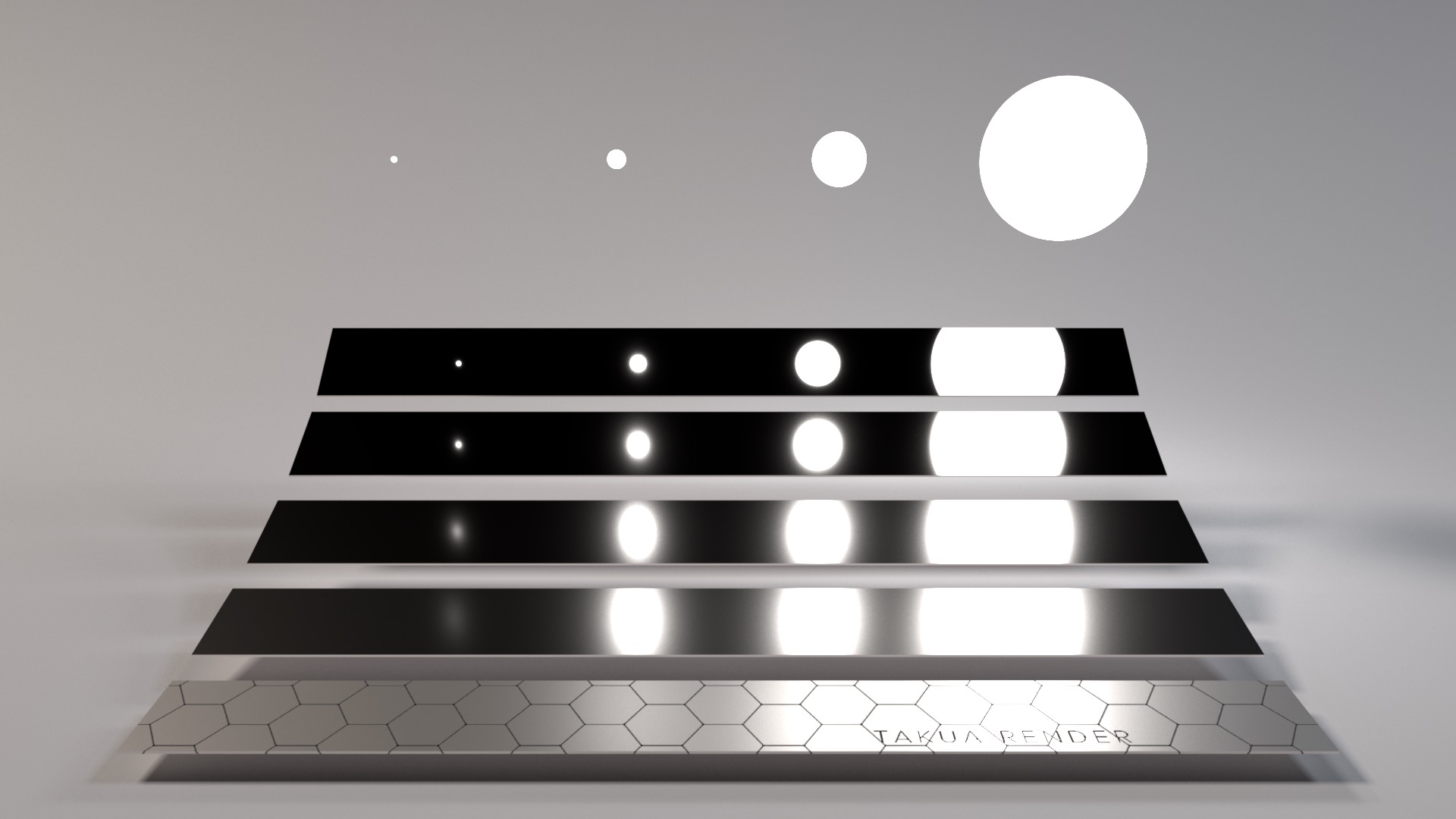The above scene is difficult to render using any single path sampling technique because of the various combinations of surface glossiness and emitter size/intensity. For large emitter/low gloss combinations, importance sampling by the BSDF tends to result in lower variance. In the case, the reason is that a given random ray direction is more likely to hit the large light than it is to fall within a narrow BSDF lobe, so matching the sample distribution to the BSDF lobe is more efficient. However, for small emitter/high gloss combinations, the reverse is true. If we take the standard Veach scene and sample by only BSDF and then only by light source, we can see how each strategy fails in different cases. Both of these renders would eventually converge if left to render for long enough, but the rate of convergence in difficult areas would be extremely slow: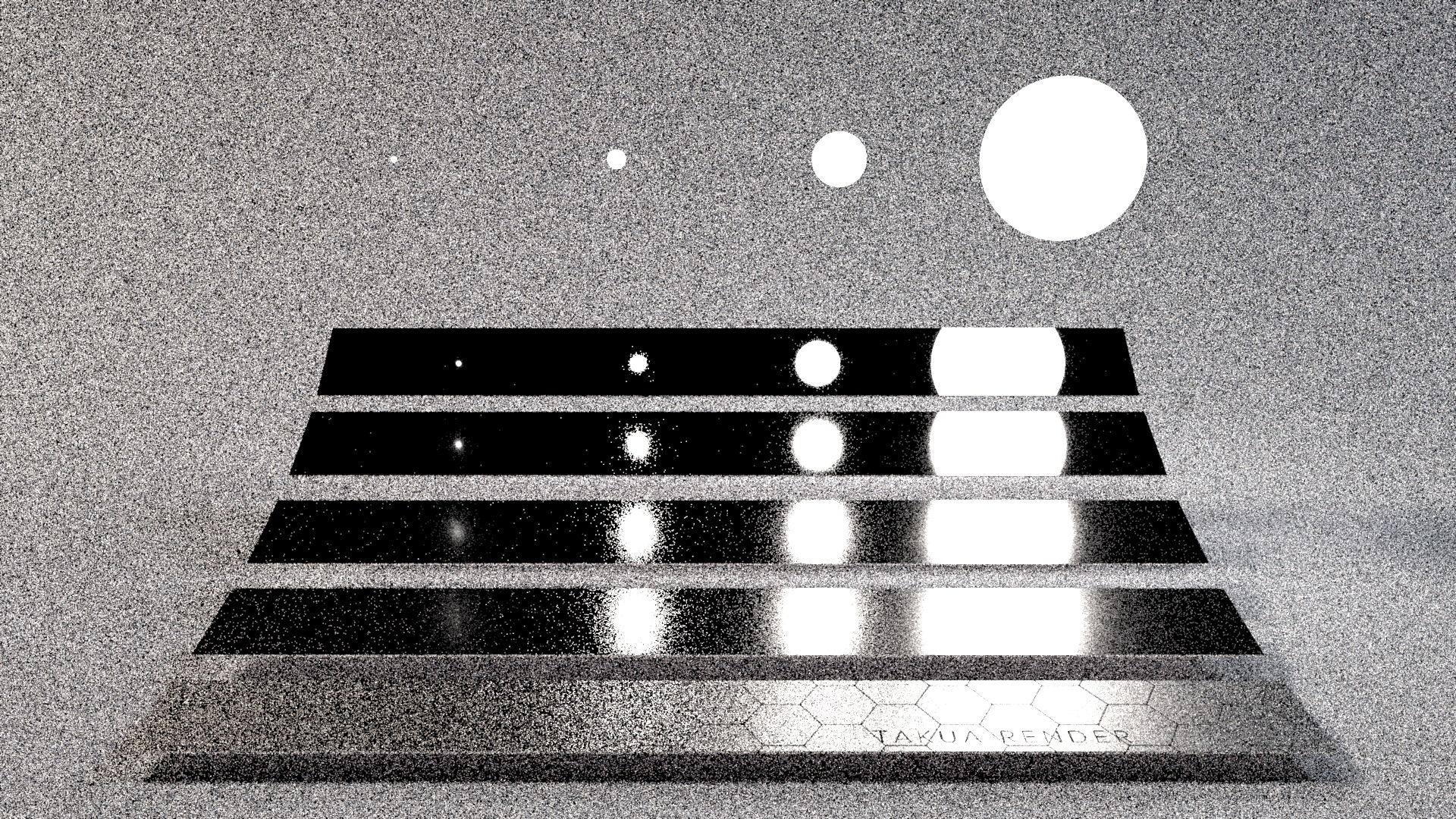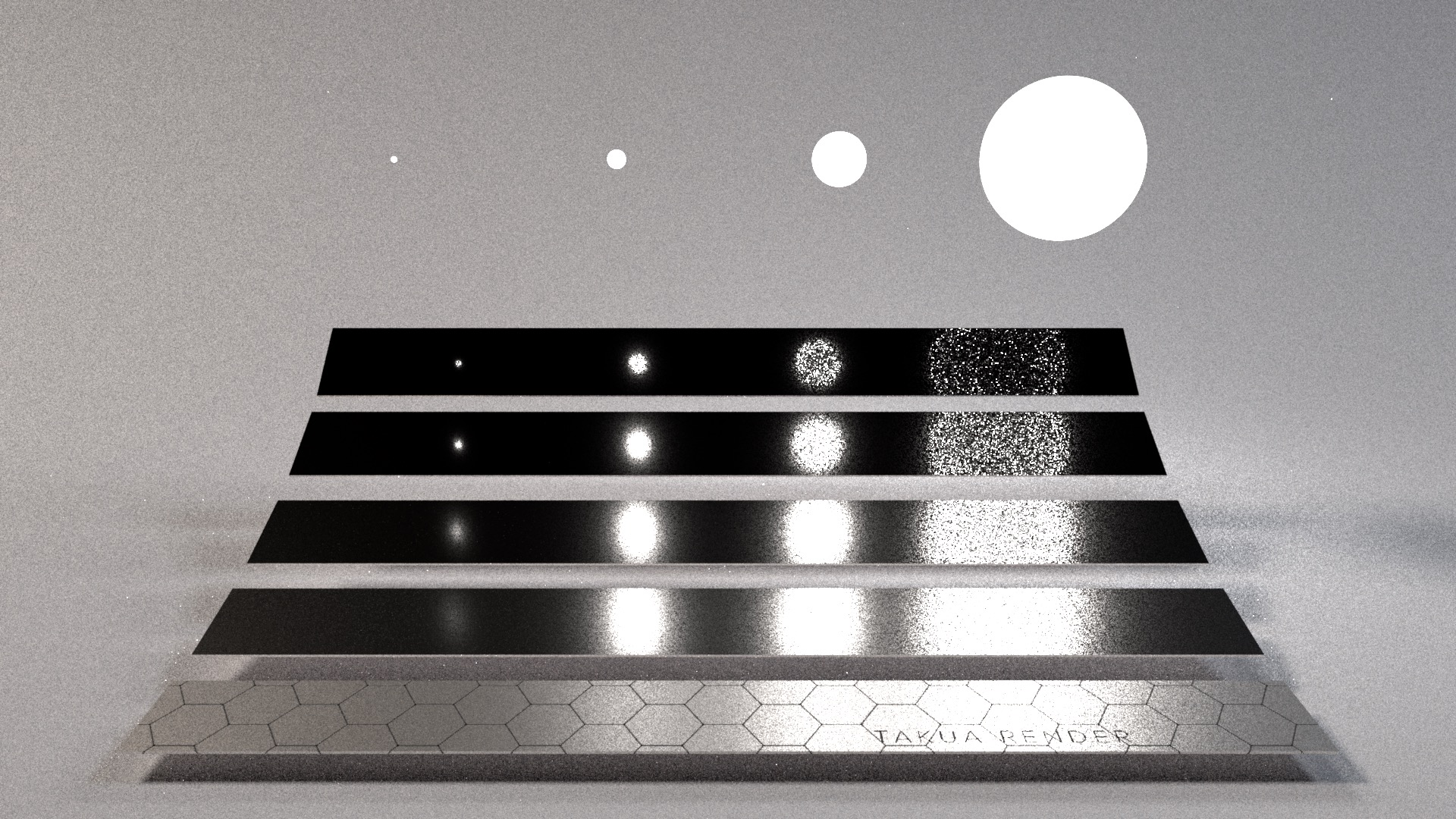MIS allows us to combine m different sampling strategies to produce a single unbiased estimator by weighting each sampling strategy by its probability distribution function (pdf). Mathematically, this is expressed as:

$\langle I_{j} \rangle_{MIS} = \sum_{i=1}^{m} \frac{1}{n_{i}} \sum_{j=1}^{n_{i}} w_{i}(X_{i,j}) \frac{f(X_{i,j})}{p_{i}(X_{i,j})}$

where Xi,j are independent random variables drawn from some distribution function pi and wi(Xi,j) is some heuristic for weighting each sampling technique with respect to pdf. The reason MIS is able to significantly lower variance is because a good MIS weighting function should dampen contributions with low pdfs. The Veach thesis presents two good weighting heuristics, the power heuristic and the balance heuristic. The power heuristic is defined as:

$w_{i}(x) = \frac{[n_{i}p_{i}(x)]^{\beta}}{\sum_{n}^{k=1}[n_{k}p_{k}(x)]^{\beta}}$

The power heuristic states that the weight for a given sampling technique should be the pdf of the sampling technique raised to a power β divided by the sum of the pdfs of all considered sampling techniques, with each sampling technique also raised to β. For the power heuristic, β is typically set to 2. The balance heuristic is simply the power heuristic for β=1. In the vast majority of cases, the balance heuristic is a near optimal weighting heuristic (and the power heuristic can cover most remaining edge cases), assuming that the base sampling strategies are decent to begin with.

For the standard Veach MIS demo scene, the best result is obtained by using MIS to combine BSDF and light sampling. The following image is the Veach scene again, this time rendered using MIS with 64 iterations. Note that all highlights are now roughly equally converged and the entire image matches the reference render above, apart from noise: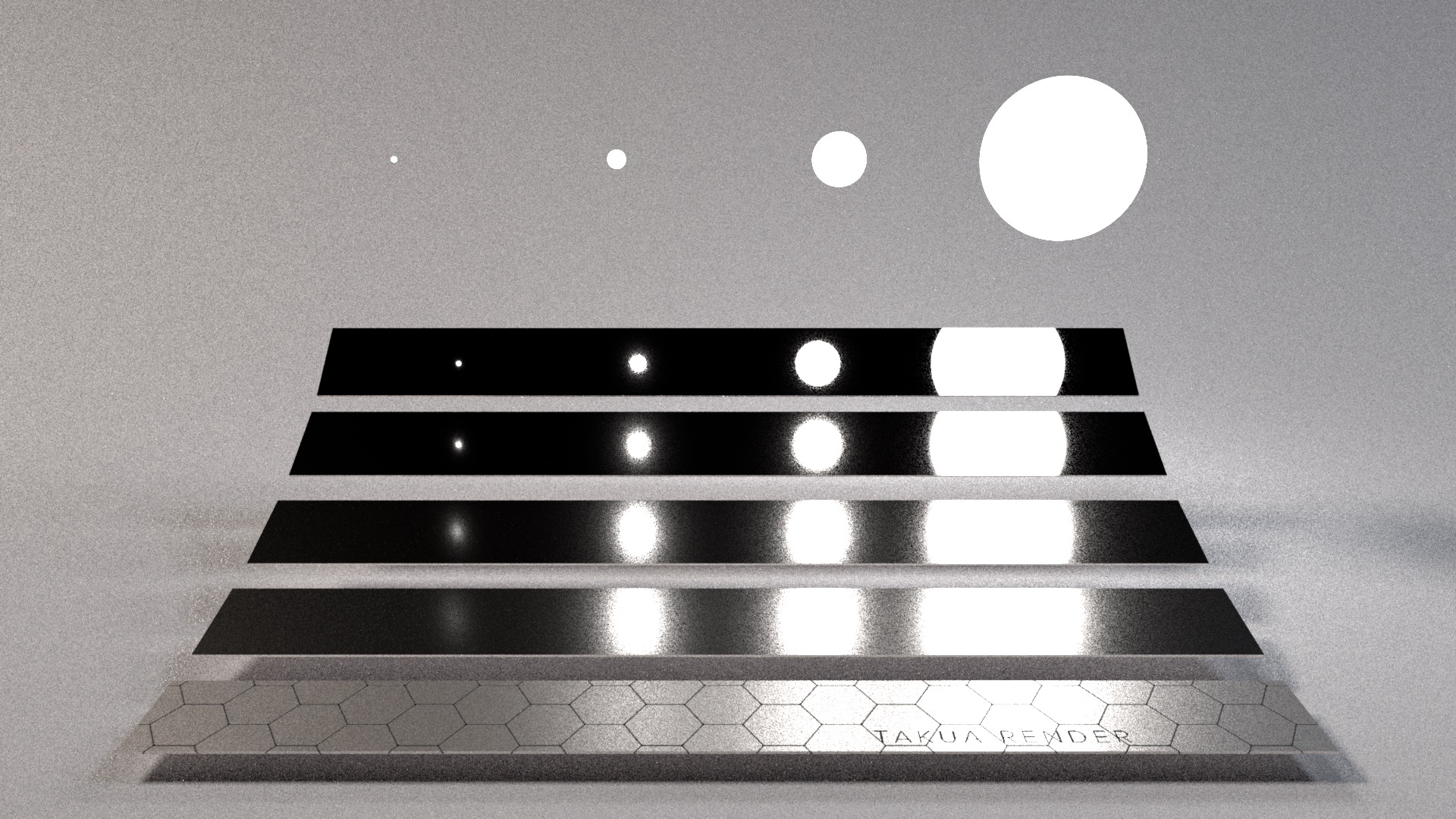BDPT inherently does not necessarily have an improved convergence rate over vanilla unidirectional pathtracing; BDPT gains its significant edge in convergence rate only once MIS is applied since BDPT’s efficiency comes from being able to extract a large number of path sampling techniques out of a single bidirectional path. To demonstrate the impact of MIS on BDPT, I rendered the following images using BDPT with and without MIS. The scene is a standard Cornell Box, but I replaced the back wall with a more complex scratched, glossy surface. The first image is the fully converged ground truth render, followed by with and without MIS: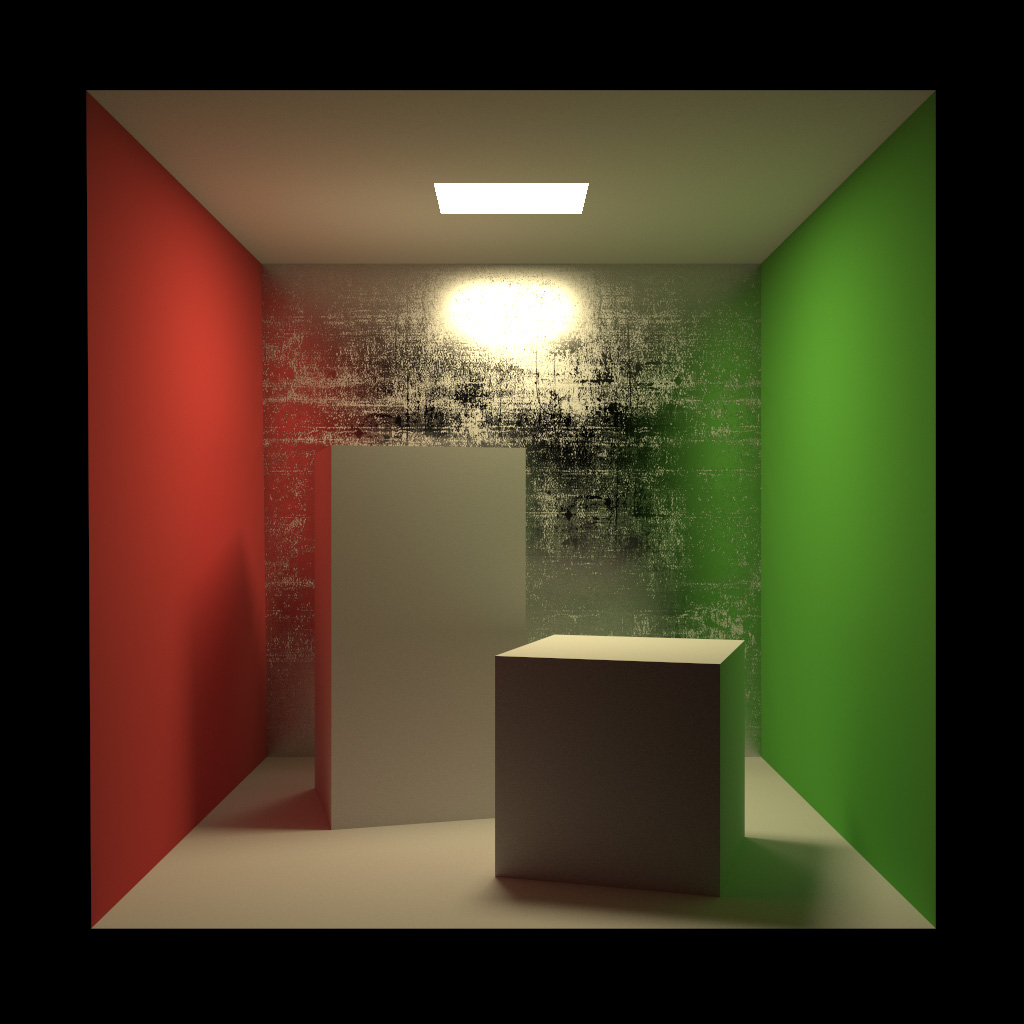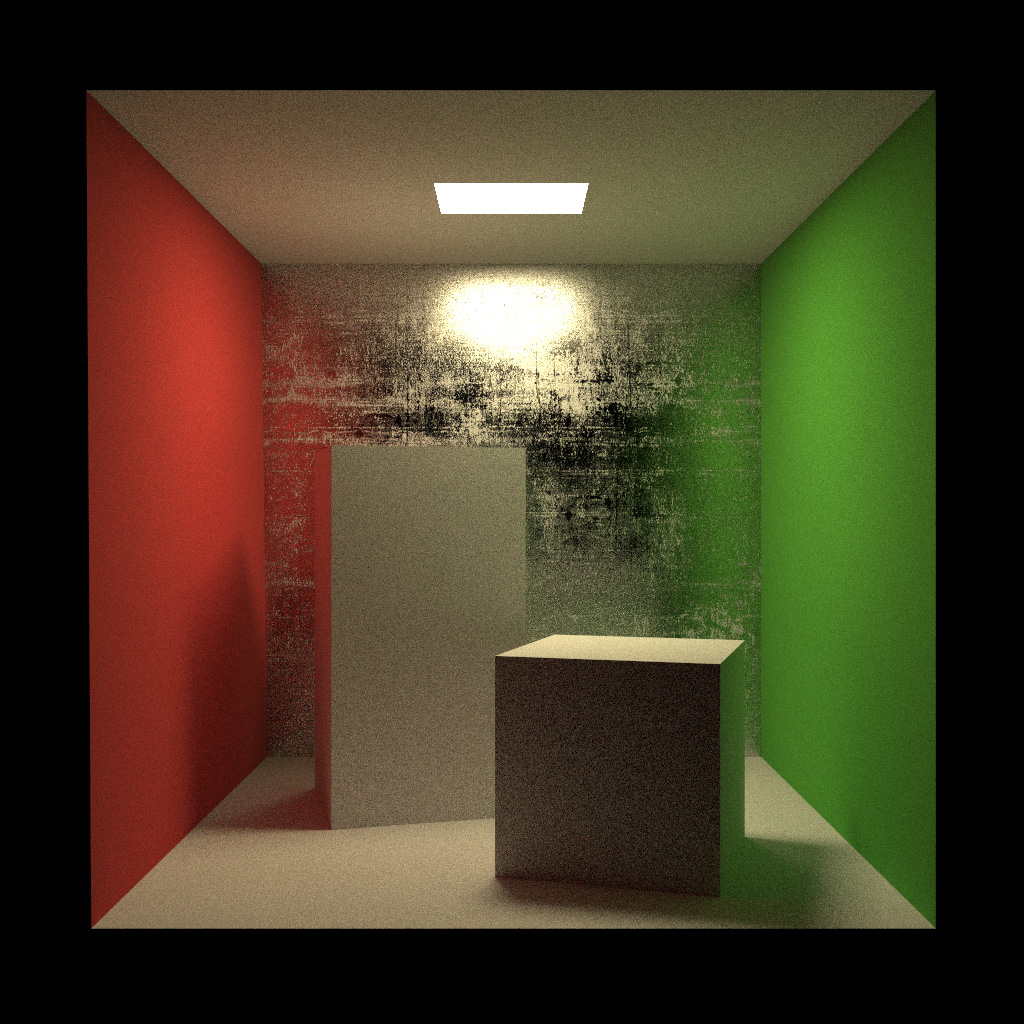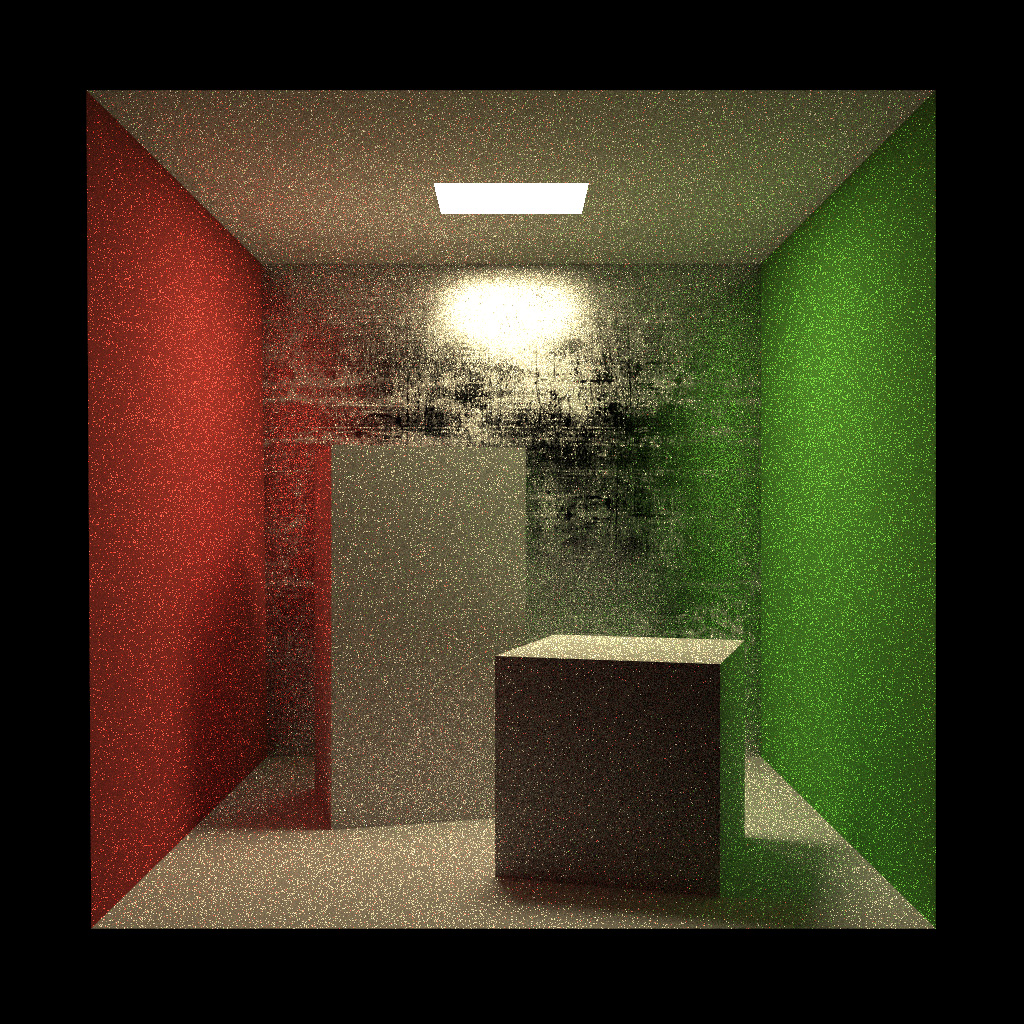As seen above, the version of BDPT without MIS is significantly less converged. BDPT without MIS will still converge to the correct solution, but in practice can often be only as good as, or worse than unidirectional pathtracing.

Later on, we’ll discuss MIS beyond bidirectional pathtracing. In fact, MIS is the critical component to making VCM possible!

Addendum 01/12/2018: A reader noticed some brightness inconsistencies in the original versions of Figures 2 and 3, which came from bugs in Takua’s light sampling code without MIS at the time. I have replaced the original versions of Figures 1, 2, 3, and 4 with new, correct versions rendered using the current version of Takua as of the time of writing for this addendum.

Because of how much noise there is in Figures 2 and 3, seeing that they converge to the reference image might be slightly harder. To make the convergence clearer, I rendered out each sampling strategy using 1024 samples per pixel, instead of just 64: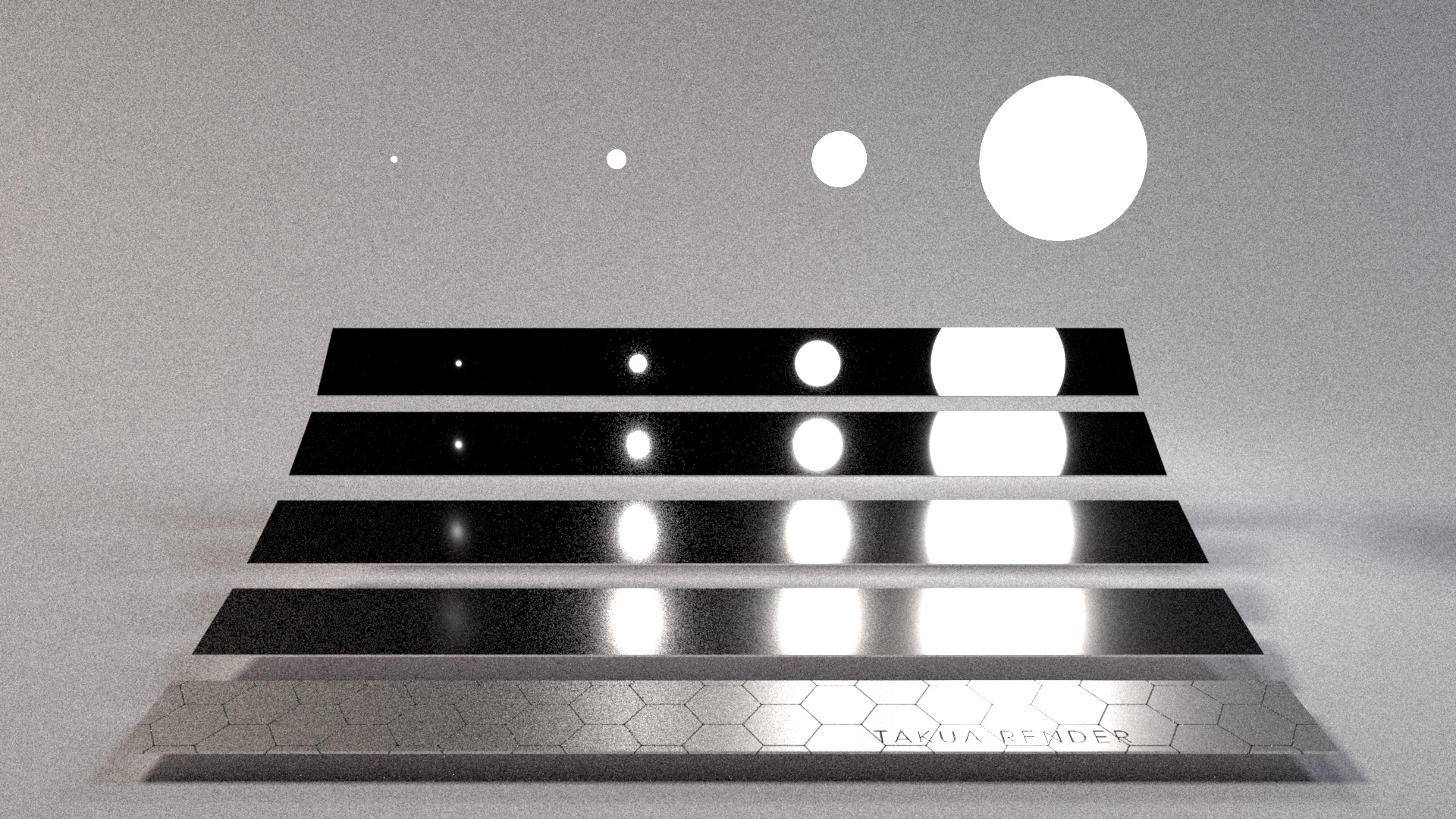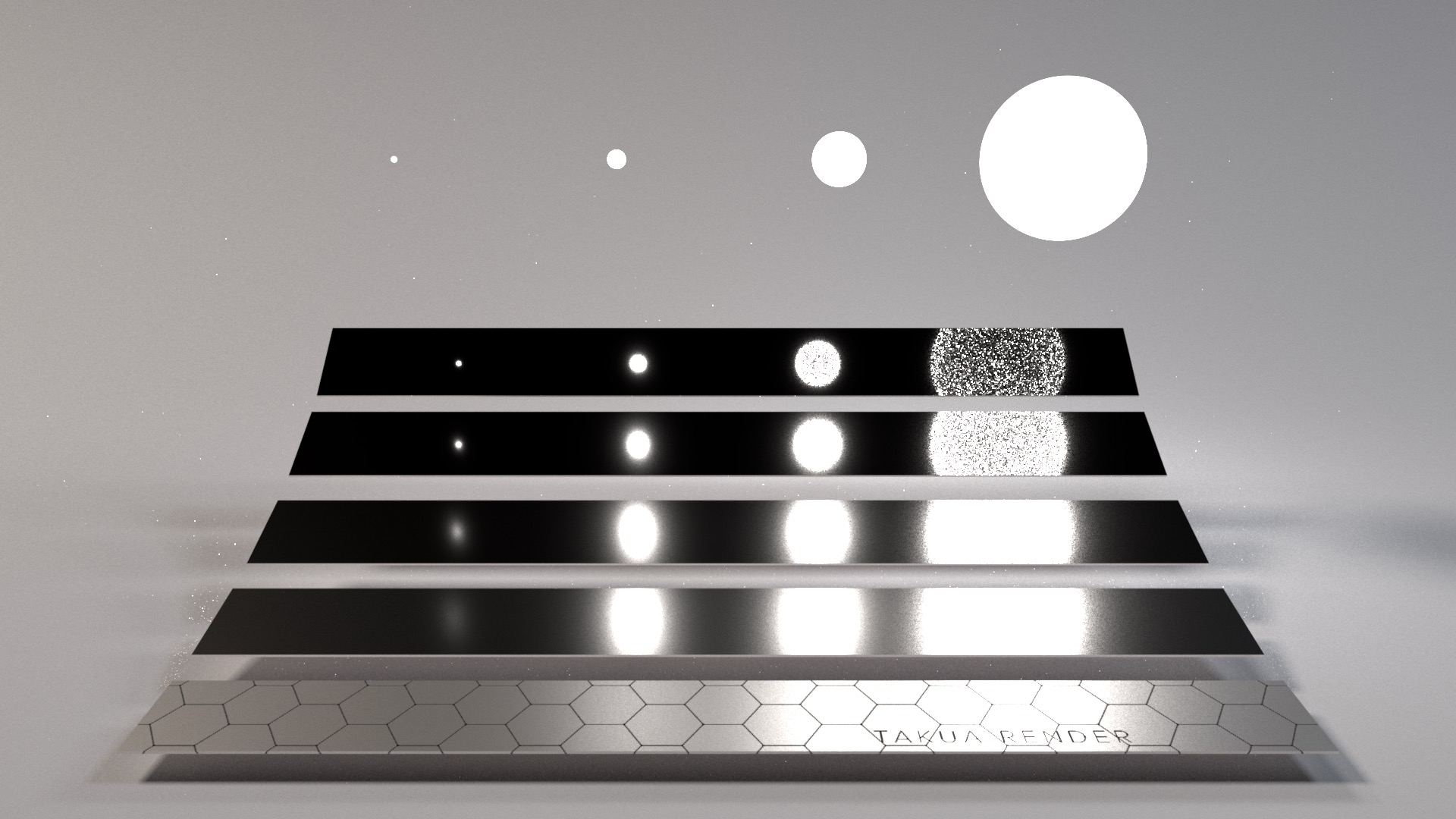Note how Figures 8 and 9 match Figure 1 exactly, aside from noise. In Figure 9, the reflection of the right-most sphere light on the top-most bar is still extremely noisy because of the extreme difficulty of finding a random light sample that happens to produce a valid bsdf response for the near-perfect specular lobe.

One last minor note: I’m leaving the main text of this post unchanged, but the updated renders use Takua’s modern shading system instead of the old one from 2015; in the new shading system, the metal bars use roughness instead of gloss, and use GGX instead of a normalized Phong variant.